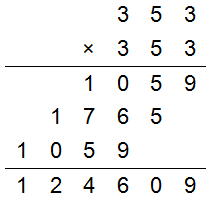# Cryptarithms

 No. of Players: 1+ Type of Game: written What you need: pen and paper

## Goal

To create or solve mathematical puzzles where digits are replaced by letters.

## How to play

A cryptarithm (or alphametic) puzzle is a puzzle where words are arranged in a mathematical expression with letters substituted for numbers. The most common mathematical expression is addition, but subtraction, multiplication and division problems can be turned into a cryptarithm by replacing numbers with appropriate letters. A few rules are usually established, such as: the leading letter cannot be zero, each letter must represent a unique digit, and solutions must be unique. Players can challenge themselves by trying to create their own cryptarithm, or they can try solving cryptarithms created by others.

## Examples

(1) Here is a famous cryptarithm and its solution:Solution:(2) Can you determine the value of each letter in this well-known multiplication cryptarithm?Solution:Home